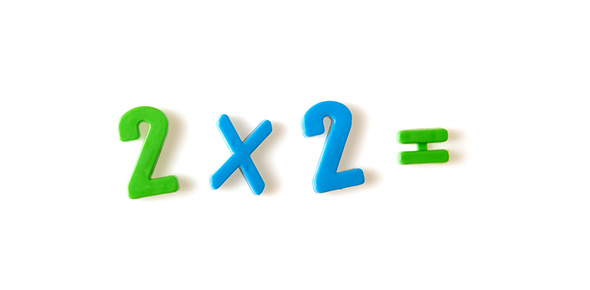# Advanced Times Table! Multiplication Trivia Test! Quiz

52 Questions | Total Attempts: 88SettingsIf you do not want to have a hard time when it comes to solving math problems involving multiplication you need to have a good understanding of the advanced times table. Do you think you do? The quiz below is a multiplication trivia test designed to test out and improve your skills, how about you give it a try and see how well you will do and if you need to practice some more.

• 1.
11 x 11 =
• 2.
11 x 12 =
• 3.
12 x 11 =
• 4.
12 x 12 =
• 5.
12 x 3 =
• 6.
12 x 4 =
• 7.
12 x 6 =
• 8.
12 x 7 =
• 9.
12 x 8 =
• 10.
12 x 9 =
• 11.
3 x 12 =
• 12.
3 x 3 =
• 13.
3 x 4 =
• 14.
3 x 6 =
• 15.
3 x 7 =
• 16.
3 x 8 =
• 17.
3 x 9 =
• 18.
4 x 12 =
• 19.
4 x 3 =
• 20.
4 x 4 =
• 21.
4 x 6 =
• 22.
4 x 7 =
• 23.
4 x 8 =
• 24.
4 x 9 =
• 25.
6 x 12 =
Related TopicsBack to top